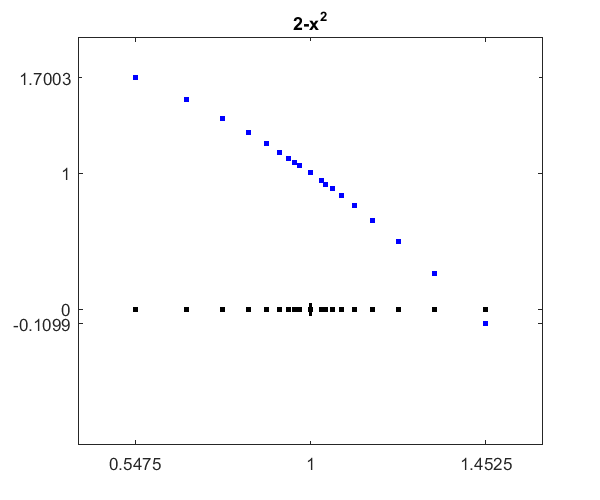# Zeroin, Part 3: MATLAB Zero Finder, FZERO

MATLAB adds capability to search for an interval with a sign change.

### Contents

#### Seeking a sign change.

We are looking for a zero of a real-valued function, $f(x)$, of a real variable, $x$. All of the versions of zeroin that I have described in the previous posts in this series, part 1 and part 2, have required a starting interval $[a,b]$ with a sign change. What if we don't know such an interval?

MathWorks added the possibility of a search for a sign change to precede the Dekker/Brent zeroin. The algorithm is straightforward. Start at a single given $x$. Take an interval centered at $x$ with half-width $x/50$. Evaluate $f(x)$ at the interval endpoints. If the signs match, increase the interval width by a factor $\sqrt{2}$ and repeat until a sign change is detected.

Here is a bit of code.

   type signchange

function [a,b] = signchange(f,x)
% SIGNCHANGE [a,b] = signchance(f,x) seeks an
% interval [a,b] where f(x) changes sign.

a = x;
b = x;
if x ~= 0
dx = x/50;
else
dx = 1/50;
end
while sign(f(a)) == sign(f(b))
dx = sqrt(2)*dx;
a = x - dx;
b = x + dx;
end
end



#### An example

Let's compute $\sqrt{2}$ by using the full-blown fzero from the MATLAB library to find a zero of $2-x^2$.

%  f = @(x) 2 - x^2;


Set a display parameter asking for intermediate results.

%  opt = optimset('display','iter');


Start the search a $x = 1$.

%  fzero(f,1,opt)


We get one line of output each time a is decreased and b is increased. All the values of f(a) are positive and all the values of f(b) are also positive until b = 1.452548 when the first sign change is reached.

%  Search for an interval around 1 containing a sign change:
%   Func-count    a          f(a)             b          f(b)        Procedure
%      1        1.000000      1.000000      1.000000      1.000000   initial interval
%      3        0.971716      1.055769      1.028284      0.942631   search
%      5        0.960000      1.078400      1.040000      0.918400   search
%      7        0.943431      1.109937      1.056569      0.883663   search
%      9        0.920000      1.153600      1.080000      0.833600   search
%     11        0.886863      1.213474      1.113137      0.760926   search
%     13        0.840000      1.294400      1.160000      0.654400   search
%     15        0.773726      1.401348      1.226274      0.496252   search
%     17        0.680000      1.537600      1.320000      0.257600   search
%     19        0.547452      1.700297      1.452548     -0.109897   search


Now the classic zeroin algorithm can reliably and rapidly find the zero.

%  Search for a zero in the interval [0.547452, 1.45255]:
%   Func-count    x          f(x)             Procedure
%     19        1.452548     -0.109897        initial
%     20        1.397600     0.0467142        interpolation
%     21        1.413990   0.000631974        interpolation
%     22        1.414214  -9.95025e-08        interpolation
%     23        1.414214   7.86149e-12        interpolation
%     24        1.414214  -4.44089e-16        interpolation
%     25        1.414214  -4.44089e-16        interpolation
%
%  Zero found in the interval [0.547452, 1.45255]
%
%  ans =
%     1.414213562373095


Here is a plot showing where the search for the sign change is carried out. The search begins in the center, expands symmetrically to the left and right, and succeeds when the negative value at the far right is discovered.

   zeroin_blog3plotPublished with MATLAB® R2015a

|

### 댓글

댓글을 남기려면 링크 를 클릭하여 MathWorks 계정에 로그인하거나 계정을 새로 만드십시오.